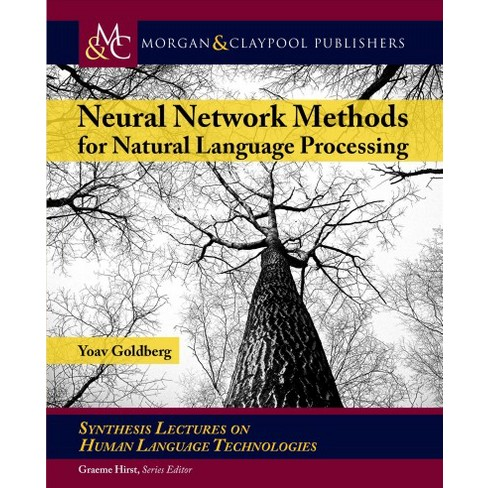# [Book] Neural Network Methods for Natural Language Processing

One of my recent reads: Neural Network Methods for Natural Language Processing.

The book is divided into four parts.

#### Part I

The book starts by a long introduction to natural language processing (NLP) and the associated linguistic tasks. This introduction presents also the typical aspects of machine learning models: losses, optimization (via stochastic gradient descent), regularization (via a norm of the parameters as an additive term to the loss function to be optimized). Then, it presents neural networks (at this stage, the Multi Layer Perceptron (MLP)) and how the linear modeling approach translates into them: Essentially, successive linear transformations of the input variables followed by a pointwise application of a non-linear function such as sigmoid, tanh, ReLU(X) := max(0, x), etc. It presents also a few tricks specific to neural networks such as the dropout technique (randomly removing some connections between layers), and a few specific problems such as vanishing (think tanh gradients for big input values) and exploding gradients or dead neurons (think outputs of a ReLU for negative values).

#### Part II

This part of the book deals with how to go from the machine learning tools to NLP solutions of the typical tasks (e.g. Part-of-speech tagging (POS), named-entity recognition (NER), chunking, syntactic parsing). It starts by explaining which linguistic features are important for textual data, and from there feature functions are `manually’ designed. This corresponds essentially to the pre-deep learning approach: handcrafting of features. Note that these handcrafted features can be fed into classical ML models as well as neural networks.

On the language modeling task (predicting the distribution of the next word given the sequence of previous words), the author illustrates the shortcomings of the classical approach:

• Use of the Markov assumption;

• very large and sparse input space that grows exponentially with the size of the lookback window.

Neural networks are a potential solution to these two problems:

• Use a recurrent neural networks to obtain an `infinite’ lookback window,

• use distributed representations (e.g. word embeddings) to share statistical properties across `close’ vocabulary and ngrams.

For me, the books really starts at Chapter 9. where the neural networks are introduced as a good alternative to solve the language modeling problem. Then follows, a couple of chapters on the word embeddings and how it relates to the word-context matrices (count-based methods) and their factorization. Goldberg showed in his papers the link between distributional (count-based) and distributed representations.

#### Part III

This part of the book tackles the `specialized architectures’. This is the main and most interesting part. It can be viewed as a good introduction to recurrent neural networks (RNN) (from simple RNN to custom architectures leveraging bi-LSTMs) and 1D convolutional neural networks (CNN) in the context of NLP, i.e. ngrams and gappy-ngrams (aka skip-grams) extractors and embedders. From Chapter 16, the book is more or less a literature review. What’s nice here is that the author rewrites the contributions and models of the literature papers in his own set of notations. The consistent use of notations and terminology makes it easy to read unlike the unhomogeneous literature. Basically, in these chapters we learn to stack different bi-LSTMs and combine different networks (viewed as computational modules) by concatenation or sum/average in a continuous bag-of-words (CBOW) fashion. The pinnacle of the presented models is the sequence-to-sequence RNN (implemented using a bi-LSTM) with attention. Attention is a method to allow the model to select its most relevant inputs, i.e. it can fit a weighted sum of its input so that it eases its learning. Besides the better results, it provides a bit of interpretability by looking at the weighting at a given step in the sequence.

#### Part IV

A collection of more advanced topics:

• Recursive neural networks for trees;

• Structured output prediction (Adapting the standard CRF to work with bi-LSTMs; Note that this model is state-of-the-art for many tagging problems);

• Cascaded, multi-task and semi-supervised learning (basically, plugging networks (or only outputs) into one another (e.g. (pre-trained or not) word embeddings). One can benefit from shared parameters (less data greedy), more supervision signals by leveraging other tasks and their datasets, some regularization as well as one can try to build a model that works well on many tasks, etc.

I think this book is a good read: From the very basic and old school to the recent developments. It is totally hype free, and the author highlights when the models fall short. Even for people having a good knowledge of the field, it can be interested as a reference, and for the fact that all models are written with the same terminology and unified set of notations which is quite clear.

It’s a bit unfortunate however to notice so many typos, especially in the final chapters. I hope that the next edition will be properly edited. It would also be nice to have a GitHub repo associated to the book and containing the implementation of the presented models in a common style.